Refer to our Texas Go Math Grade 3 Answer Key Pdf to score good marks in the exams. Test yourself by practicing the problems from Texas Go Math Grade 3 Lesson 15.2 Answer Key Draw Quadrilaterals.

Unlock the Problem

Connect You have learned to classify quadrilaterals by the number of pairs of opposite sides that are parallel, by the number of pairs of sides of equal length, and by the number of right angles.

Activity 1

Use grid paper to draw quadrilaterals.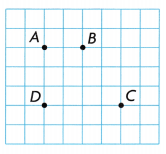Materials; ruler

• Use a ruler to draw line segments from points A to B, from B to C, from C to D, and from D to A.Trapezoid
Explanation:
exactly 1 pair of opposite sides that are parallel lengths of sides could be the same

Activity 2

Draw a figure that does not belong.
Materials; ruler

A. Here are three examples of a parallelogram. Draw an example of a quadrilateral that is not a parallelogram.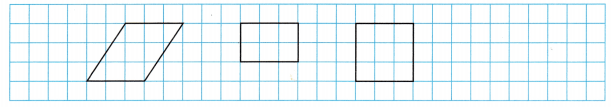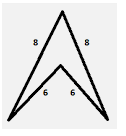Explanation:
The above shape is not a parallelogram
A Square has 2 pairs of opposite sides that are parallel
4 sides that are of equal length
4 right angles

B. Here are three examples of a rectangle. Draw a quadrilateral that is not a rectangle.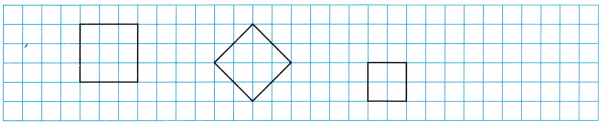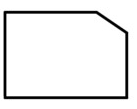Explanation:
The above figure is not a rectangle
A Square has 2 pairs of opposite sides that are parallel
4 sides that are of equal length
4 right angles

C. Here are three examples of a rhombus. Draw a quadrilateral that is not a rhombus.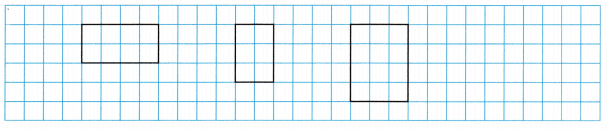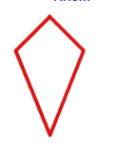Explanation:
A rhombus 2 pairs of opposite sides that are parallel
4 sides that are of equal length

D. Here are three examples of a rhombus. Draw a quadrilateral that is not a rhombus.Explanation:
A rhombus 2 pairs of opposite sides that are parallel
4 sides that are of equal length

Math Talk
Mathematical Processes

Compare your drawings with your classmates. Explain how your drawings are alike and how they are different.
Answer: Some are same and some are different
Explanation:
When compared the drawings with my friend some of them are same
And some of them are different

Share and Show

Question 1.
Choose four endpoints that connect to make a rectangle.Think: A rectangle has 2 pairs of opposite sides that are parallel, 2 pairs of sides of equal length, and 4 right angles.Explanation:
A rectangle has 2 pairs of opposite sides that are parallel,
2 pairs of sides of equal length, and 4 right angles.

Question 2.
4 right anglesName ____________Explanation:
The Above figure has 4 right angles

Question 3.
2 pairs of opposite sides that are parallel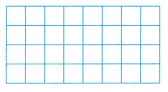Name _____________Explanation:
2 pairs of opposite sides that are parallel

Draw a quadrilateral that does not belong. Then explain why.

Question 4.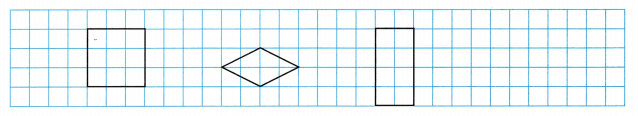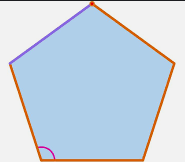Explanation:
The above figure is not a quadrilateral
The figure is a polygon
A polygon has 5 sides

Problem Solving

Question 5.
Multi-Step Amy has 4 straws of equal length. Name the quadrilaterals she can make
using these 4 straws. ____________
Amy cuts one of the straws in half. She uses the two halves and two of the other straws to make a quadrilateral. Name a quadrilateral she can make using these 4 straws. ____________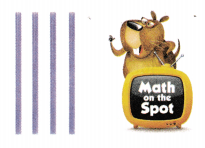and Rectangle
Explanation:
Amy has 4 straws of equal length. The quadrilaterals she can make
using these 4 straws is square
Amy cuts one of the straws in half.
She uses the two halves and two of the other straws to make a quadrilateral.
A quadrilateral she can make using these 4 straws is a rectangle

Fill in the bubble for the correct answer choice.

Question 6.
Reasoning Clara is building a frame for a doghouse wall using four wooden boards. Two of the boards are the same length. Each of the other two boards is a different length. What shape can she build?
(A) rectangle
(B) square
(C) trapezoid
(D) rhombus
Explanation:
Clara is building a frame for a doghouse wall using four wooden boards.
Two of the boards are the same length.
Each of the other two boards is a different length.
The shape must be a Trapezoid.

Question 7.
Use Diagrams The pieces of a pattern for a mosaic are drawn on grid paper. The pattern shows four quadrilaterals. Which quadrilateral is not a parallelogram?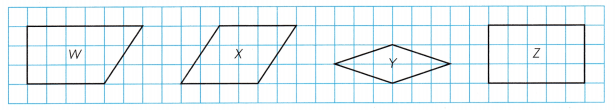(A) Shape W
(B) Shape Y
(C) Shape X
(D) Shape Z
Explanation:
Shape W is not a parallelogram
It is a Trapezoid.

Question 8.
Multi-Step Ethan wants the figure on the grid paper to be a parallelogram. At which point should he place the fourth vertex?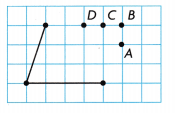(A) Point D
(B) Point B
(C) Point C
(D) Point A
Explanation:
Ethan wants the figure on the grid paper to be a parallelogram.
he has to place the fourth vertex at point B

Texas Test Prep

Question 9.
Jordan drew a quadrilateral with 2 pairs of opposite sides that are parallel. Which figure could NOT be the quadrilateral Jordan drew?Explanation:
Option D is not a parallelogram
It is a Trapezoid.

### Texas Go Math Grade 3 Lesson 15.2 Homework and Practice Answer Key

Question 1.
2 pairs of opposite sides that are parallel and no right anglesName _____________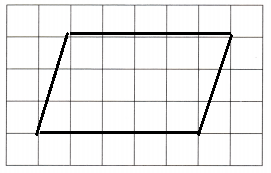Explanation:
A Parallelogram has 4 Sides
2 opposite sides are parallel to each other
No right angles

Question 2.
1 pair of opposite sides that are parallel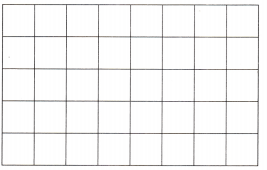Name _____________Explanation:
exactly 1 pair of opposite sides that are parallel lengths of sides could be the same

Question 3.
Draw a quadrilateral that does not belong. Then explain why.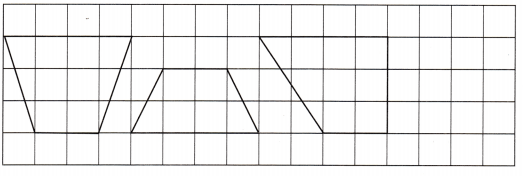Explanation:
The square does not belongs to the group
because the remaining are trapezoids

Problem Solving

Question 4.
Enrique has the craft sticks shown. Name the possible polygons Enrique can make.Explanation:
They can draw a trapezoid,
because, only one pair of sides are parallel
the other two sticks are different sizes

Lesson Check

Question 5.
Midori uses wooden sticks to make a quadrilateral. She has 2 equal-length long sticks and 2 equal-length short sticks. If she places the sticks so there are 4 right angles, which figure does Midori make?
(A) rhombus
(B) rectangle
(C) square
(D) trapezoid
Explanation:
Midori uses wooden sticks to make a quadrilateral.
She has 2 equal-length long sticks and 2 equal-length short sticks.
If she places the sticks so there are 4 right angles,
Midori make a rectangle.

Question 6.
Han has 2 long pencils of equal length and 2 short pencils of equal length. If he arranges them so that the long pencils and short pencils are opposite and parallel, which figure does he make?
(A) trapezoid
(B) rhombus
(C) square
(D) parallelogram
Explanation:
Han has 2 long pencils of equal length and 2 short pencils of equal length.
If he arranges them so that the long pencils and short pencils are opposite and parallel,
It makes a parallelogram

Question 7.
Rex draws four quadrilaterals on grid paper. Which quadrilateral is NOT a rhombus?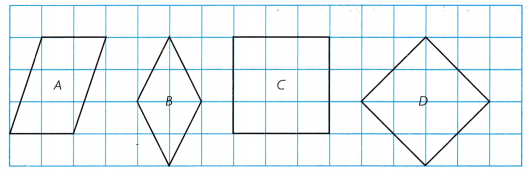(A) Shape A
(B) Shape B
(C) Shape C
(D) Shape D
Explanation:
Shape A is not a Rhombus
But it is a parallelogram.

Question 8.
Multi-Step Gigi wants to make a figure with four craft sticks. She has the three craft sticks shown and needs one more. Which size of craft stick does Gigi need to make the figure?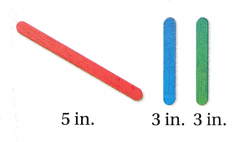(A) 5-in. craft stick; rectangle
(B) 3-in. craft stick; rectangle
(C) 5-in. craft stick; square
(D) 6-in. craft stick; square